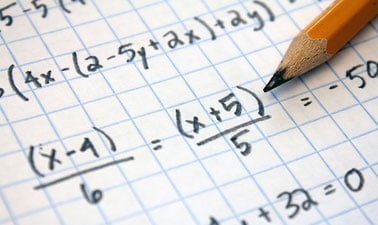# Doane University: College Algebra

This College Algebra course will cover fundamental concepts of algebra required to interpret a variety of functions and equations.

This course is eligible for Audit and Verified Certificate only.8 weeks
8–12 hours per week
Self-paced
Free

This College Algebra course will cover fundamental concepts of algebra required to interpret a variety of functions and equations. Topics within this course include: linear, quadratic, polynomial, rational, exponential, inverse functions and their graphs, linear inequalities, and linear systems of equations. Students who successfully complete this course will demonstrate increased ability in problem-solving and logical thinking.

### At a glance

• Institution: DoaneX
• Subject: Math
• Level: Intermediate
• Prerequisites:
None
• Language: English
• Video Transcript: English
• Associated skills: Problem Solving, Auditing, Algebra, Linear Systems

# What you'll learn

Skip What you'll learn

As a student in this course, students will:

• Compute with and categorize Real numbers
• Solve and apply equations and inequalities
• Analyze and use graphs and functions
• Solve systems of equations and inequalities
• Compute with and factor polynomials and polynomial functions
• Compute with rational expressions and solve rational equations
• Compute with and simplify radicals, roots, and complex numbers
• Solve and graph quadratic functions